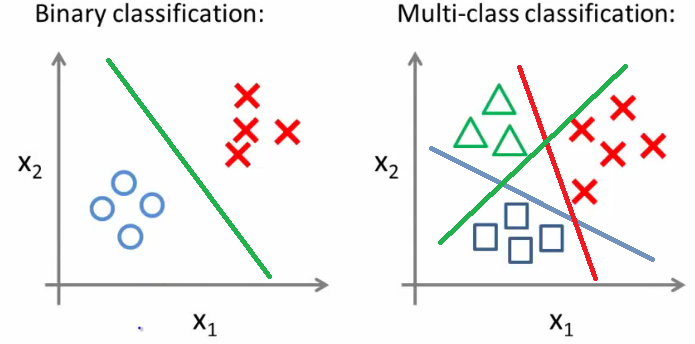#In the previous story we talked about Linear Regression for solving regression problems in machine learning, This story we will talk about Logistic Regression for classification problems.

You may be wondering why the name says regression if it is a classification algorithm, well,It uses the regression inside to be the classification algorithm.

Classification : Separates the data from one to another. This story we talk about binary classification ( 0 or 1). Here target variable is either 0 or 1. Goal is to find that green straight line (which separates the data at best). So we use regression for drawing the line, makes sense right?We only accept the values between 0 and 1 (We don’t accept other values) to make a decision (Yes/No). There is an awesome function called Sigmoid or Logistic function, we use to get the values between 0 and 1. This function squashes the value (any value) and gives the value between 0 and 1.

So far we know that we first apply the linear equation and apply Sigmoid function for the result so we get the value which is between 0 and 1. The hypothesis for Linear regression is h(X) = θ0+θ1*X.

How does it work?

1. First we calculate the Logit function: logit = θ0+θ1*X (hypothesis of linear regression)
2. We apply the above Sigmoid function (Logistic function) to logit.
3. We calculate the error, Cost function (Maximum log-Likelihood).
4. Next step is to apply Gradient descent to change the θ values in our hypothesis.

We got the Logistic regression ready, we can now predict new data with the model we just built.

Views: 1061

Tags: logistic regression

Comment

Join Data Science Central